•5星
127KB lunzilx 2021-01-11 16:02:37
•5星
108.34MB guoruibin123 2021-04-27 06:03:44
• 4KB wangmu7206 2019-04-21 17:17:45
• 51单片机交通信号灯系统设计程序 51单片机

46KB weixin_44042579 2020-12-21 11:33:56
• 273KB weixin_43532704 2021-03-04 10:28:00
• 1.1MB qq_38639612 2021-07-21 11:19:08
• 34KB weixin_38621427 2020-07-17 15:23:07
• 2.24MB lu_shuang 2014-10-11 09:55:16
•5星
13KB u011738275 2013-08-19 08:54:37
• 基于51单片机模拟交通灯程序.rar 51单片机

25KB weiweiweiIT 2020-02-17 23:11:05
• 1.29MB baidu_28455067 2017-12-02 13:22:43
• 10.37MB qq_43372640 2019-07-11 16:17:49
• 基于51单片机的交通信号灯设计 c语言 单片机

基于51单片机交通信号灯设计 这里写目录标题基于51单片机交通信号灯设计摘要：一．前言二、实验设计目的三．设计思路四．硬件介绍4.1定时器/计数器4.2定时器／计数器的概念4.3定时器／计数器的相关寄存器4.5...

摘要：

基本要求：

• （1）利用单片机令十字路口的红绿灯交替点亮和熄灭。
• （2）可通过按键对红绿灯点亮和熄灭时间进行调整及设置。
• （3）能对红绿灯点亮和熄灭时间进行倒计时时间显示。
提高部分
• （4）可进行紧急或特殊情况的人工处理控制。（例如救护车或消防车）
• （5）有内部24小时钟，可根据时间表对对红绿灯点亮和熄灭时间进行自动调整。
展开全文weixin_44908159 2021-08-21 18:29:51
•5星
389KB suiyiwuhui 2011-12-26 20:34:03
• 用51单片机实现交通信号灯 单片机

51单片机实现交通信号灯1、新建工程项目及文件1.1 新建工程1.2 新建文件2、新建protues电路2.1 新建工程2.2 选择元器件2.3 连接元器件3、导入文件并仿真3.1 导入文件3.2 开始仿真 1、新建工程项目及文件 1.1 新建...

1、新建工程项目及文件

1.1 新建工程

1.1.1新建工程项目1.1.2选择保存路径以及工程名称1.1.3选择51芯片选择AT89C51
工程即可创建完成

1.2 新建文件

1.2.1新建文件1.2.2将代码写入保存
代码如下

#include "reg52.h"
#define dm P0
typedef unsigned int u16;
typedef unsigned char u8;

sbit ISA=P3^5;
sbit ISB=P3^6;
sbit ISC=P3^7;
sbit k1=P3^4;
sbit fmq=P3^3;

u8 code smgduan={0x3f,0x06,0x5b,0x4f,0x66,0x6d,0x7d,0x07,0x7f,0x6f};
//u8 code smgduan={0x6f,0x7f,0x07,0x7d,0x6d,0x66,0x4f,0x5b,0x06,0x3f};
u8  smgdata;
u8 second=0;

void delay(u16 i)
{
while(i--);
}

void Time01Init()
{
TMOD|=0X11;
TH0=0XFC;
TL0=0X18;
ET0=1;
TH1=0XFF;
TL1=0X9C;
ET1=1;
EA=1;
}

void SmgDisplay()
{
u8 i;
for(i=0;i<8;i++)
{
switch(i)
{
case(0):
ISA=0;ISB=0;ISC=0; break;
case(1):
ISA=1;ISB=0;ISC=0; break;
case(2):
ISA=0;ISB=1;ISC=0; break;
case(3):
ISA=1;ISB=1;ISC=0; break;
case(4):
ISA=0;ISB=0;ISC=1; break;
case(5):
ISA=1;ISB=0;ISC=1; break;
case(6):
ISA=0;ISB=1;ISC=1; break;
case(7):
ISA=1;ISB=1;ISC=1; break;
}
dm=smgdata[i];
delay(100);
dm=0x00;
}
}

sbit	p1_0=P1^0;
sbit	p1_1=P1^1;
sbit	p1_2=P1^2;
sbit	p1_3=P1^3;
sbit	p1_4=P1^4;
sbit	p1_5=P1^5;
sbit	p1_6=P1^6;
sbit	p1_7=P1^7;
sbit	p2_0=P2^0;
sbit	p2_1=P2^1;
sbit	p2_2=P2^2;
sbit	p2_3=P2^3;

void deng()
{
if(second==56)
{
second=0;
}
if(second<25)
{
smgdata=smgduan[(25-second)/10%10]; //Î÷ÂÌ25-01
smgdata=smgduan[(25-second)/1%10];

smgdata=smgduan[(28-second)/10%10];//±±ºì28-03
smgdata=smgduan[(28-second)/1%10];

smgdata=smgduan[(25-second)/10%10];//¶«ÂÌ25-01
smgdata=smgduan[(25-second)/1%10];

smgdata=smgduan[(28-second)/10%10];//ÄÏºì28-03
smgdata=smgduan[(28-second)/1%10];
SmgDisplay();
p1_0=0;
p1_1=0;
p1_2=1;
p1_3=0;
p1_4=0;
p1_5=1;
p1_6=1;
p1_7=0;
p2_0=0;	//8
p2_1=1;	//9
p2_2=0;	//10
p2_3=0;	//11
}
else if(second<28)
{
smgdata=smgduan[(28-second)/10%10];//Î÷»Æ03-01
smgdata=smgduan[(28-second)/1%10];

smgdata=smgduan[(28-second)/10%10];//±±ºì03-01
smgdata=smgduan[(28-second)/1%10];

smgdata=smgduan[(28-second)/10%10];//¶«»Æ03-01
smgdata=smgduan[(28-second)/1%10];

smgdata=smgduan[(28-second)/10%10];//ÄÏºì03-01
smgdata=smgduan[(28-second)/1%10];
SmgDisplay();
p1_0=0;
p1_1=1;
p1_2=0;
p1_3=0;
p1_4=1;
p1_5=0;
p1_6=1;
p1_7=0;
p2_0=0;	//8
p2_1=1;	//9
p2_2=0;	//10
p2_3=0;	//11
}
else if(second<53)
{
smgdata=smgduan[(56-second)/10%10];//Î÷ºì28-03
smgdata=smgduan[(56-second)/1%10];

smgdata=smgduan[(53-second)/10%10];//±±ÂÌ25-01
smgdata=smgduan[(53-second)/1%10];

smgdata=smgduan[(56-second)/10%10];//¶«ºì28-03
smgdata=smgduan[(56-second)/1%10];

smgdata=smgduan[(53-second)/10%10];//ÄÏÂÌ25-01
smgdata=smgduan[(53-second)/1%10];
SmgDisplay();
p1_0=1;
p1_1=0;
p1_2=0;
p1_3=1;
p1_4=0;
p1_5=0;
p1_6=0;
p1_7=0;
p2_0=1;	//8
p2_1=0;	//9
p2_2=0;	//10
p2_3=1;	//11 */
}
else if(second<56)
{
smgdata=smgduan[(56-second)/10%10];//Î÷ºì05-01
smgdata=smgduan[(56-second)/1%10];

smgdata=smgduan[(56-second)/10%10];//±±»Æ05-01
smgdata=smgduan[(56-second)/1%10];

smgdata=smgduan[(56-second)/10%10];//¶«ºì05-01
smgdata=smgduan[(56-second)/1%10];

smgdata=smgduan[(56-second)/10%10]; //ÄÏ»Æ05-01
smgdata=smgduan[(56-second)/1%10];
SmgDisplay();
p1_0=1;
p1_1=0;
p1_2=0;
p1_3=1;
p1_4=0;
p1_5=0;
p1_6=0;
p1_7=1;
p2_0=0;	//8
p2_1=0;	//9
p2_2=1;	//10
p2_3=0;	//11*/
}
}

void main()
{
while(1)
{
Time01Init();
//key();
TR0=1;
TR1=0;
deng();

}
}

void Time0() interrupt 1
{
static u16 i;
TH0=0XFC;
TL0=0X18;
i++;
if(i==1000)	//1s==1000ms
{
i=0;
second++;
}
}

保存文件并选择路径编辑文件名点击保存即可
1.2.3添加文件到工程并编译文件1.2.4 生成hex文件点击ok再次编译即可生成

2、新建protues电路

2.1 新建工程

2.1.1新建工程2.1.2编辑文件名称及路径点击下一步直至完成即可

2.2 选择元器件

2.2.1添加元器件
打开元器件库并选择需要的元器件2.3 连接元器件

将元器件按图示连接3、导入文件并仿真

3.1 导入文件

3.1.1导入
双击51芯片并导入之前生成的hex文件添加完成后点击确定

3.2 开始仿真

点击开始仿真结果如图总结：通过本次用51单片机实现交通信号灯，简单的了解了51单片机的应用。也通过本次仿真，了解到硬件编程和软件所不同的区别。

展开全文qq_45999753 2020-10-08 16:51:57
• 270KB qq_61928686 2021-10-09 19:56:58
• 391KB chenlu0528 2021-10-06 21:06:15
• 391KB qq_61928686 2021-10-09 19:56:57
• c语言控制51单片机完成交通信号灯（红绿灯） c语言 51单片机

每隔7秒开始改变信号灯， 一个方向红灯亮，一个方向绿灯亮，绿灯变红灯时要先闪烁6次，变黄灯，再变红灯。 红灯直接变绿灯。 流程图如下 电路图如下 本实验主要通过定时器来完成闪烁和计时功能，主函数用来设置和...

完成目标：
模拟十字路口（东南西北走向）交通灯：通过单片机的P1口控制实验箱上6个LED灯（两红两绿两黄），按照自定义规则控制交通灯。
要求有如下过程：某种颜色灯转另一种颜色的灯之前，要闪烁六次。

我的程序自定义规则如下：
每隔7秒开始改变信号灯，
一个方向红灯亮，一个方向绿灯亮，绿灯变红灯时要先闪烁6次，变黄灯，再变红灯。
红灯直接变绿灯。
流程图如下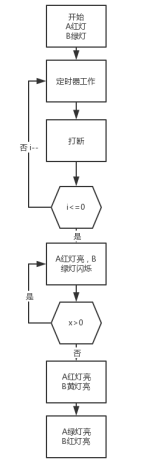电路图如下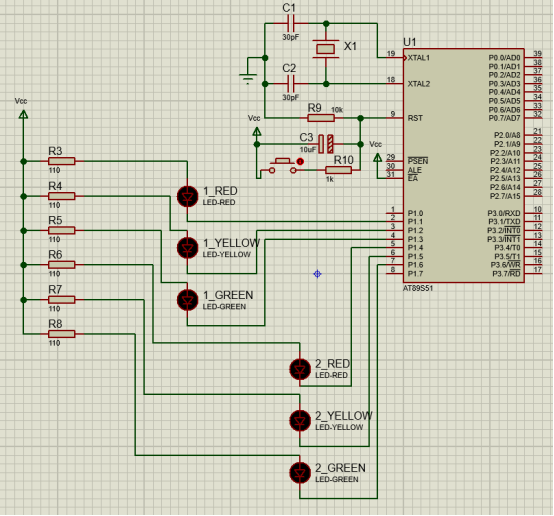本实验主要通过定时器来完成闪烁和计时功能，主函数用来设置和启动定时器

void main()
{
TMOD=0x01;		//开启定时器
TH0=0x00;
TL0=0x00;
EA=1;			//允许中断
ET0=1;			//to中断
TR0=1;			//启动T0
P1=0x3c;		//A路口红灯亮，B路口绿灯亮
while(1);		//等待中断
}

下面是定时器部分，用于完成信号灯的转换任务

void timer0() interrupt 1
{
TH0=0x00;
TL0=0x00;
i--;
if(i<=0)
{
i=100;
if(P1==0x3c) //判断路口灯亮情况
{
for(x=6;x>0;x--) //B路口绿灯闪烁
{
P1=0x7c;
delay1(1);
P1=0x3c;
delay1(1);
}
P1=0x5c;	  //B路口黄灯亮。绿灯灭
delay1(4);
P1=0x66;	  //A路口绿灯亮，B路口红灯亮
}
else
{
for(x=6;x>0;x--)//A路口绿灯闪烁
{
P1=0x6e;
delay1(1);
P1=0x66;
delay1(1);
}
P1=0x6a;	 //A路口黄灯亮，绿灯灭
delay1(4);
P1=0x3c;	//A路口红灯亮，B路口绿灯亮
}
}
}

完整Proteus电路和c语言程序都可以下载，本人使用keil编程，将工程文件中的.hex文件按调入proteus电路的单片机中，运行程序即可看到实验效果。

展开全文weixin_46523923 2021-11-29 15:07:43
• 项目实战：51单片机控制交通信号灯 PROTEUS 和51单片机教程（附仿真文件+源代码） 单片机 交通信号灯 PROTEUS

51单片机控制交通信号灯 说明：最近接了一个小项目，要在一个十字路口用单片机实现交通信号灯的控制 我试着用仿真软件做了一下，效果还可以，希望和大家一起学习 总体设计仿真图 单片机仿真设计部分 公路信号灯显示...

51单片机控制交通信号灯
说明：最近接了一个小项目，要在一个十字路口用单片机实现交通信号灯的控制

我试着用仿真软件做了一下，效果还可以，希望和大家一起学习

总体设计仿真图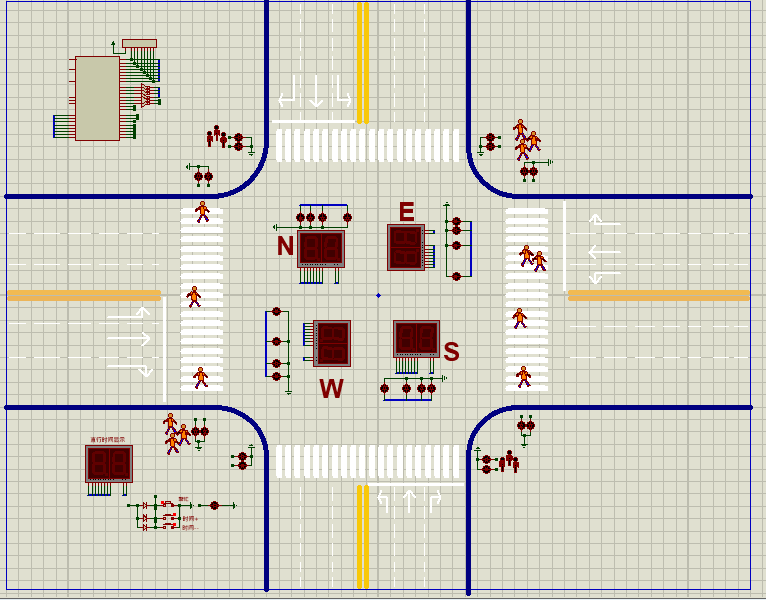单片机仿真设计部分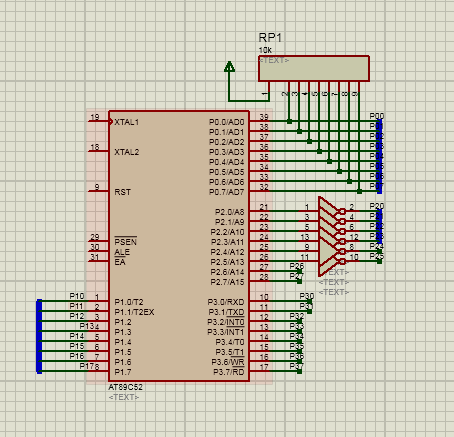公路信号灯显示仿真部分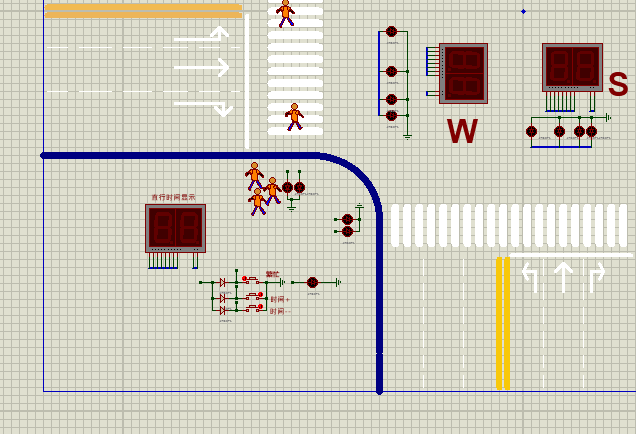四个方向仿真设计部分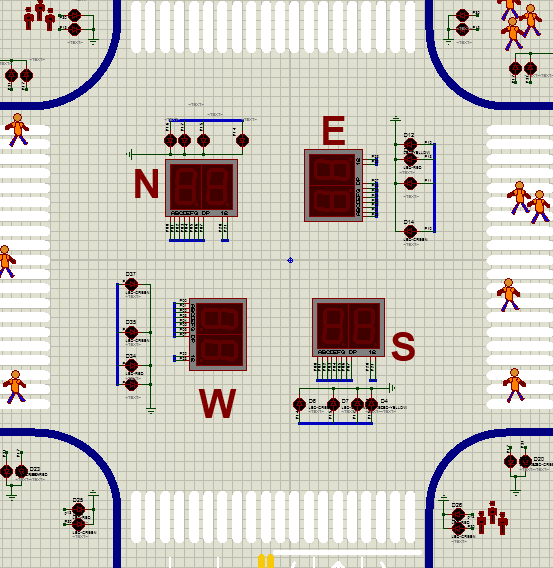启动仿真后开始计时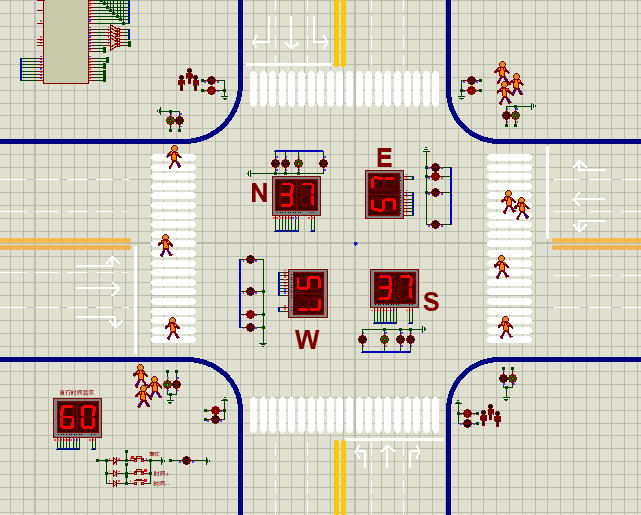C语言参考源代码：

/*****************************************************
十字路口交通灯控制 C 程序

******************************************************/

#define uchar unsigned char
#define uint unsigned int
#include <reg52.h>
/定义控制位*****************/
sbit Time_Show_LED2=P2^5;//Time_Show_LED2控制位
sbit Time_Show_LED1=P2^4;//Time_Show_LED1控制位
sbit EW_LED2=P2^3; //EW_LED2控制位
sbit EW_LED1=P2^2; //EW_LED1控制位
sbit SN_LED2=P2^1; //SN_LED2控制位
sbit SN_LED1=P2^0; //SN_LED1控制位
sbit SN_Yellow=P1^6;//SN黄灯
sbit EW_Yellow=P1^2;//EW黄灯
sbit EW_Red=P1^3;//EW红灯
sbit SN_Red=P1^7;//SN红灯
sbit EW_ManGreen=P3^0;//EW人行道绿灯
sbit SN_ManGreen=P3^1;//SN人行道绿灯
sbit Special_LED=P2^6;//交通正常指示灯
sbit Busy_LED=P2^7;//交通繁忙指示灯
sbit Nomor_Button=P3^5;//交通正常按键
sbit Busy_Btton=P3^6;//交通繁忙按键
sbit Special_Btton=P3^7;//交通特殊按键
sbit Reduces_Button=P3^4;//时间减
bit Flag_SN_Yellow; //SN黄灯标志位
bit Flag_EW_Yellow;//EW黄灯标志位
char Time_EW;//东西方向倒计时单元
char Time_SN;//南北方向倒计时单元
uchar EW=60,SN=40,EWL=19,SNL=19; //程序初始化赋值，正常模式
uchar EW1=60,SN1=40,EWL1=19,SNL1=19;//用于存放修改值的变量
uchar code table={0x3F,0x06,0x5B,0x4F,0x66,0x6D,0x7D,0x07,0x7F,0x6F};//1~~~~9段选码
uchar code S={0X28,0X48,0X18,0X48,0X82,0X84,0X81,0X84};//交通信号灯控制代码
/延时子程序**/
void Delay(uchar a)
{
uchar i;
i=a;
while(i–){;}
}
/显示子函数*********/
void Display(void)
{
char h,l;
h=Time_EW/10;
l=Time_EW%10;
P0=table[l];
EW_LED2=1;
Delay(2);
EW_LED2=0;
P0=table[h];
EW_LED1=1;
Delay(2);
EW_LED1=0;

h=Time_SN/10;
l=Time_SN%10;
P0=table[l];
SN_LED2=1;
Delay(2);
SN_LED2=0;
P0=table[h];
SN_LED1=1;
Delay(2);
SN_LED1=0;

h= EW1/10;
l= EW1%10;
P0=table[l];
Time_Show_LED1=1;
Delay(2);
Time_Show_LED1=0;
P0=table[h];
Time_Show_LED2=1;
Delay(2);
Time_Show_LED2=0;
}
/外部0中断服务程序**/

void EXINT0(void)interrupt 0 using 1
{
EX0=0; //关中断

{
EW1+=5;
SN1+=5;
if(EW1>=100)
{
EW1=99;
SN1=79;
}
}
if(Reduces_Button
0) //时间减
{
EW1-=5;
SN1-=5;
if(EW1<=40)
{
EW1=40;
SN1=20;
}

}

if(Nomor_Button0)//测试按键是否按下，按下为正常状态
{
EW1=60;
SN1=40;
EWL1=19;
SNL1=19;
Busy_LED=0;//关繁忙信号灯
Special_LED =0;//关特殊信号灯
}
if(Busy_Btton
0) //测试按键是否按下，按下为繁忙状态
{
EW1=45;
SN1=30;
EWL1=14;
SNL1=14;
Special_LED=0;//关特殊信号灯
Busy_LED=1;//开繁忙信号灯

}
if(Special_Btton==0)//测试按键是否按下，按下为特殊状态
{
EW1=75;
SN1=55;
EWL1=19;
SNL1=19;
Busy_LED=0;//关繁忙信号灯
Special_LED =1;//开特殊信号灯

}
EX0=1;//开中断
}
/***T0中断服务程序/
void timer0(void)interrupt 1 using 1
{
static uchar count;
TH0=(65536-50000)/256;
TL0=(65536-50000)%256;
count++;

if(count==10)
{

if(Flag_SN_Yellow1) //测试南北黄灯标志位
{SN_Yellow=~SN_Yellow;}
if(Flag_EW_Yellow
1) //测试东西黄灯标志位
{EW_Yellow=~EW_Yellow;}
}
if(count20)
{
Time_EW–;
Time_SN–;
if(Flag_SN_Yellow
1)//测试南北黄灯标志位
{SN_Yellow=~SN_Yellow;}
if(Flag_EW_Yellow==1)//测试东西黄灯标志位
{EW_Yellow=~EW_Yellow;}
count=0;
}

}
/主程序开始*/
void main(void)

{
Busy_LED=0;
Special_LED=0;
IT0=1; //INT0负跳变触发
TMOD=0x01;//定时器工作于方式1
TH0=(65536-50000)/256;//定时器赋初值
TL0=(65536-50000)%256;
EA=1; //CPU开中断总允许
ET0=1;//开定时中断
EX0=1;//开外部INTO中断
TR0=1;//启动定时

while(1)

{ /S0状态***/
EW_ManGreen=0; //EW人行道禁止
SN_ManGreen=1;//SN人行道通行
Flag_EW_Yellow=0; //EW关黄灯显示信号
Time_EW=EW;
Time_SN=SN;
while(Time_SN>=5)
{P1=S; //SN通行，EW红灯
Display();}
/S1状态***/
P1=0x00;
while(Time_SN>=0)
{Flag_SN_Yellow=1; //SN开黄灯信号位
EW_Red=1; //SN黄灯亮，等待左拐信号，EW红灯

Display();
}
/S2状态***/
Flag_SN_Yellow=0; //SN关黄灯显示信号
Time_SN=SNL;
while(Time_SN>=5)
{P1=S;//SN左拐绿灯亮，EW红灯
Display();}

/S3状态***/
P1=0x00;
while(Time_SN>=0)
{Flag_SN_Yellow=1; //SN开黄灯信号位
EW_Red=1; //SN黄灯亮,等待停止信号，EW红灯

Display();}

/*赋值/
EW=EW1;
SN=SN1;
EWL=EWL1;
SNL=SNL1;

/S4状态***/
EW_ManGreen=~EW_ManGreen;//EW人行道通行
SN_ManGreen=~SN_ManGreen;//SN人行道禁止
Flag_SN_Yellow=0; //SN关黄灯显示信号
Time_EW=SN;
Time_SN=EW;
while(Time_EW>=5)
{P1=S; //EW通行，SN红灯
Display();}
/S5状态***/
P1=0X00;
while(Time_EW>=0)
{Flag_EW_Yellow=1;//EW开黄灯信号位
SN_Red=1;//EW黄灯亮，等待左拐信号，SN红灯
Display();}
/S6状态***/
Flag_EW_Yellow=0; //EW关黄灯显示信号
Time_EW=EWL;
while(Time_EW>=5)
{P1=S;//EW左拐绿灯亮，SN红灯
Display();}

/S7状态***/
P1=0X00;
while(Time_EW>=0)
{Flag_EW_Yellow=1; //EN开黄灯信号位
SN_Red=1;//EW黄灯亮，等待停止信号，SN红灯
Display();}

/*赋值/
EW=EW1;
SN=SN1;
EWL=EWL1;
SNL=SNL1;
}

}

最后，如果有什么意见或者建议欢迎您留言给我，让我们共同学习一起进步，
如果需要 程序完整源代码和 设计文件，请在下方留言或者私信我，看到后会第一时间回复。

谢谢！

展开全文weixin_44212493 2020-02-15 15:52:17
•5星
127KB lunzilx 2021-01-11 16:02:37
• weixin_42213451 2021-05-22 01:06:53
• 单片机交通信号灯源代码 文档

15KB ghnftujhg 2019-04-11 15:21:02
• 66KB weixin_38502290 2021-01-06 13:23:10
• 82KB john913 2011-04-18 11:13:56
• 979KB weixin_38743737 2021-01-06 11:38:50
• 基于51单片机的交通灯控制设计 51单片机 proteus keil C语言

qq_40223983 2019-07-12 21:29:19
• 2.8MB qq_41684392 2020-03-28 16:35:08
• weixin_32401763 2021-05-22 00:45:32
• 73KB md55550 2020-12-29 12:12:55
• 62KB x_uhen 2018-01-17 11:30:46...